# opencv实战——机器视觉检测和计数

## 引言

😀整体思路：

1. 读取图片
2. 形态学处理（在二值化前进行适度形态学处理，效果俱佳）
3. 二值化
4. 提取轮廓（进行药片分割）
5. 获取轮廓索引，并筛选所需要的轮廓
6. 画出轮廓，显示计数

opencv实现：

int main(int argc, char** argv)
{
Mat src, src_binary,dst,src_distance;
imshow("原图片", src);
Mat kernel = getStructuringElement(MORPH_RECT, Size(16, 16), Point(-1, -1));
morphologyEx(src, dst, MORPH_OPEN, kernel);
imshow("形态学",dst);
cvtColor(dst, dst, COLOR_RGB2GRAY);
threshold(dst, src_binary, 100, 255, THRESH_OTSU);
imshow("二值化", src_binary);
vector<vector<Point>> contours;
findContours(src_binary, contours, RETR_EXTERNAL, CHAIN_APPROX_NONE, Point(0, 0));
RNG rng(12345);
double area;
Point2i PL;
for (size_t i = 0; i < contours.size(); i++)
{
area = contourArea(contours[i]);
if (area < 500)continue;
PL = contours[i].front();
Scalar color = Scalar(rng.uniform(0, 255), rng.uniform(0, 255), rng.uniform(0, 255));
drawContours(src, contours, i, color, 2, 8);
putText(src, to_string(i), PL, FONT_HERSHEY_COMPLEX, 1, color, 2);
}
imshow("计数结果", src);
waitKey(0);
return 0;
}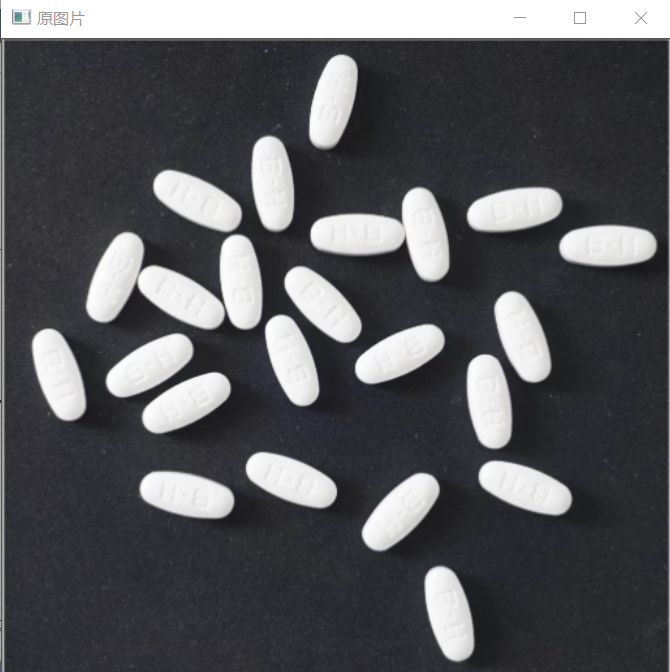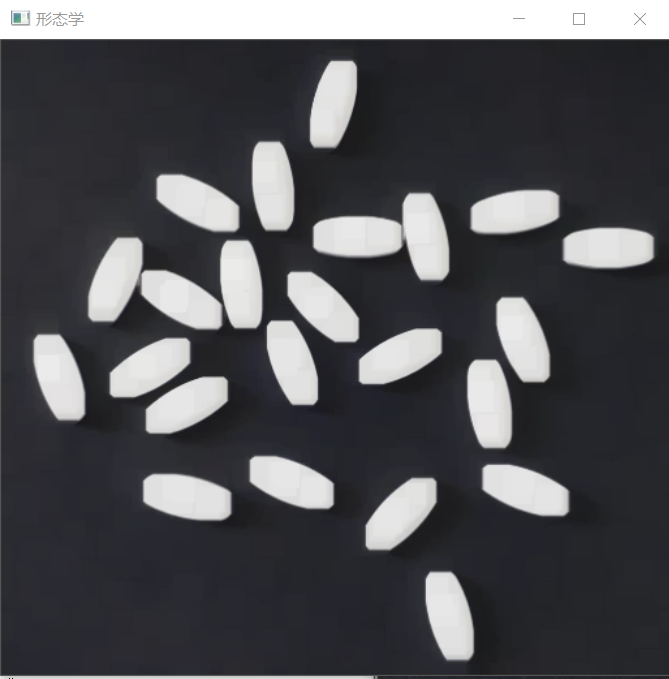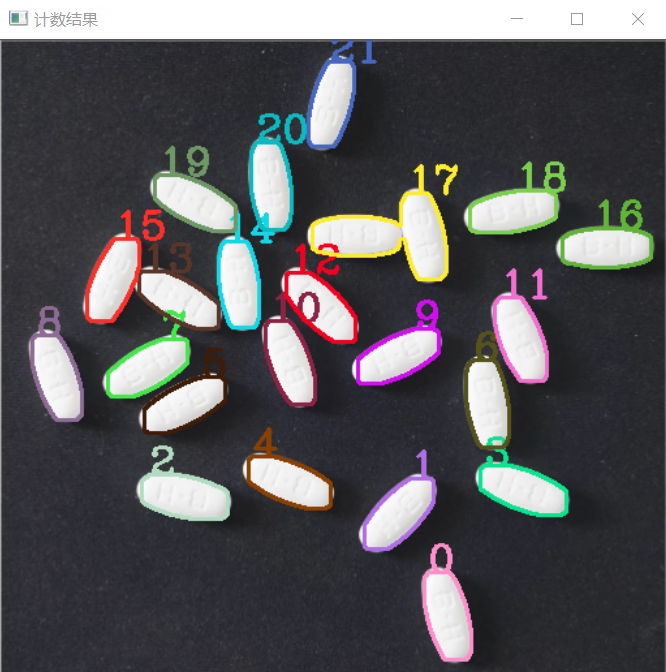由上图可以看的，原图在经过形态学处理后，可以去除很多细节，简化后续的药片分割操作。

🤨这不由得让我们思考，如果简单的形态学处理分割不了药片呢？

对于复杂的产品图片，我们可以使用基于距离变换的分水岭算法对其分割。

基于距离变换的分水岭算法检测和计数

OpenCV 采用了基于标记点的分水岭算法，在这种算法中我们要设置哪些山谷点会汇合，哪些不会。这是一种交互式的图像分割。我们要做的就是给我们已知的对象打上不同的标签（即添加注水点）。然后实施分水岭算法。每一次灌水，我们的标签就会被更新，当两个不同颜色的标签相遇时就构建堤坝，直到将所有山峰淹没，最后我们得到的边界对象（堤坝）的值为 -1。

🙁对于如何打上标签（即添加注水点）有两种办法：

opencv中，对于一张二值化的图像，后续处理方式有两种。第一种方式就是利用findContours、drawContours等函数进行轮廓分析（opencv以对轮廓的处理为主）。第二种方式就是计算连通域进行区域分析。

• 分水岭函数watershed函数原型
void watershed( InputArray image, InputOutputArray markers );

😛在执行watershed函数后，算法会根据markers传入的轮廓作为种子，对图像上其他的像素点根据分水岭算法规则进行判断，并对每个像素点的区域归属进行划定，直到处理完图像上所有像素点。而区域与区域之间的分界处的值被置为“-1”，以做区分。

•  距离变换函数distanceTransform函数原型

distanceTransform( InputArray src, OutputArray dst, int distanceType, int maskSize，int dstType = CV_32F);

🧡💛💚💙💜🤎🖤

（一）读入图像，形态学，二值化（消除噪声）

    Mat src, src_binary, dst, src_distance;
imshow("原图片", src);
Mat kernel = getStructuringElement(MORPH_RECT, Size(3, 3), Point(-1, -1));
morphologyEx(src, dst, MORPH_OPEN, kernel);
imshow("形态学", dst);
cvtColor(dst, dst, COLOR_RGB2GRAY);
threshold(dst, src_binary, 100, 255, THRESH_OTSU);
imshow("二值化", src_binary);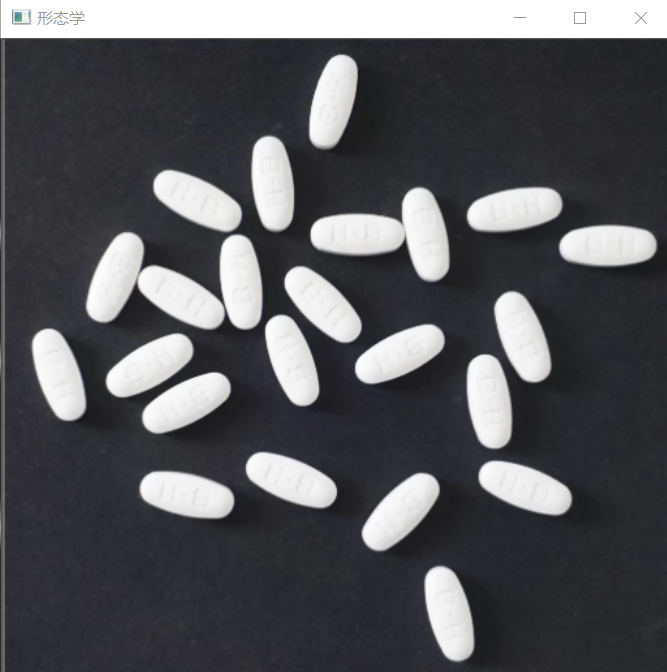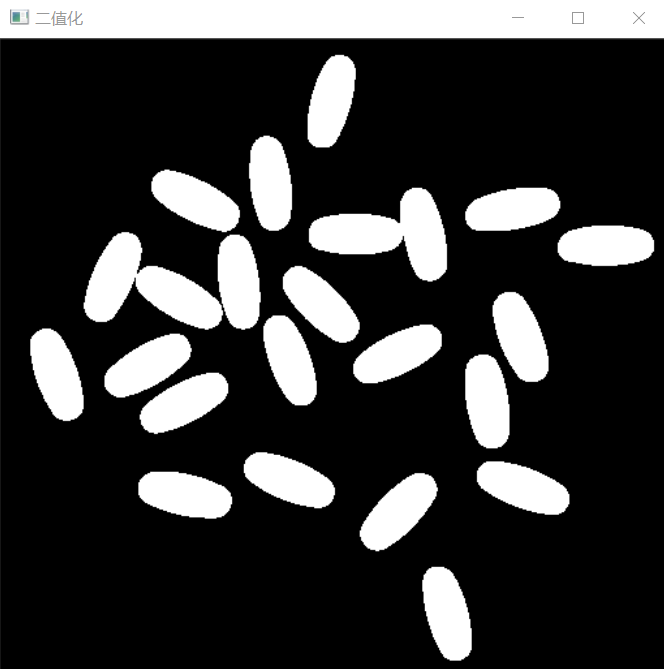（二）距离变换（归一化显示），再二值化

    distanceTransform(src_binary, src_distance, DIST_L2, 3, 5);
normalize(src_distance, src_distance, 0, 1, NORM_MINMAX);
imshow("距离变换", src_distance);
threshold(src_distance, src_distance, 0.4,1, THRESH_BINARY);
imshow("再二值化", src_distance);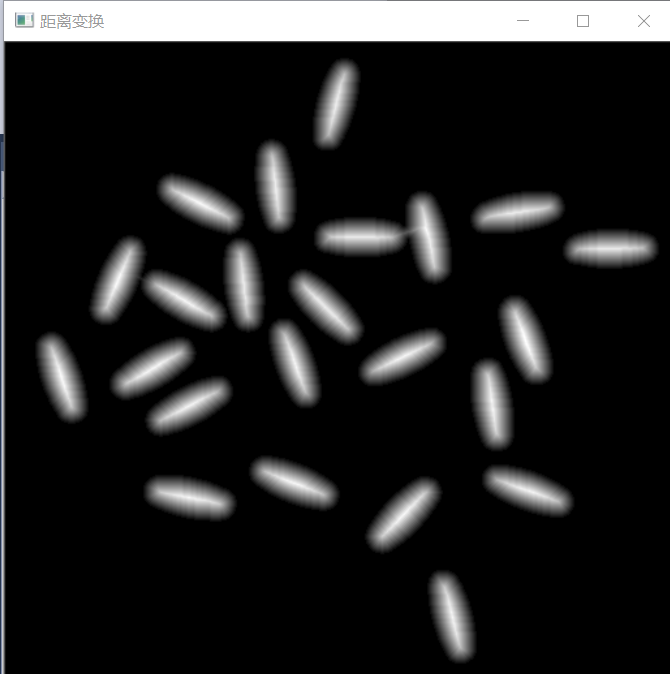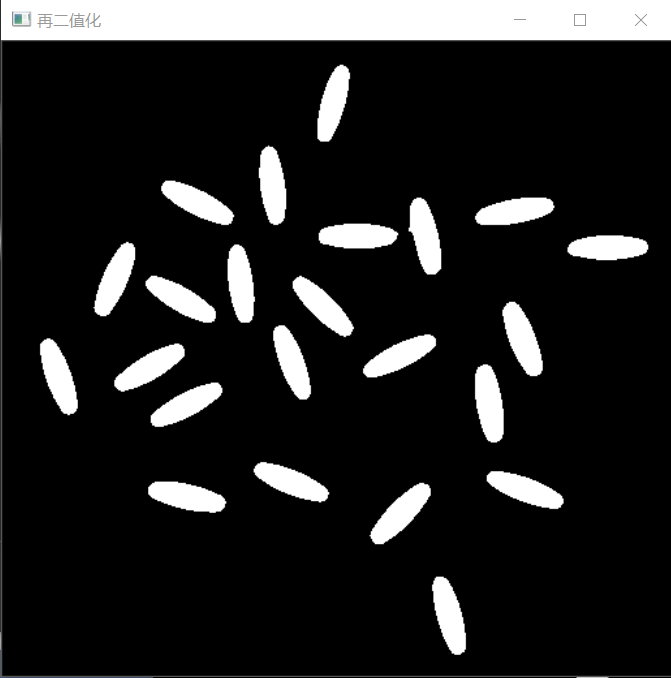（三）打上标签（添加注水点）,基于轮廓

    //寻找标记点marsk的轮廓信息 也就是分水岭的水坝
src_distance.convertTo(src_distance, CV_8UC1);
vector<vector<Point>> contours;
findContours(src_distance, contours, RETR_TREE, CHAIN_APPROX_SIMPLE);
//创建maker
Mat markers = Mat::zeros(src.size(), CV_32S);//  //因为分水岭后的边缘存储是-1，所以必须使用有符号的CV_32S
for (size_t t = 0; t < contours.size(); t++)
{
drawContours(markers, contours, static_cast<int>(t), Scalar(static_cast<int>(t) + 1), -1);//轮廓数字编号
}
circle(markers, Point(5, 5), 30, Scalar(255), -1);//关键代码（mark做一个小标记）
int index1 = 0;
//打印轮廓数据 有值的均为轮廓线
for (int row = 0; row < markers.rows; row++)
for (int col = 0; col < markers.cols; col++)
{
index1 = markers.at<int>(row, col);
cout << index1 << ",";
}

部分标签markers轮廓数据截图，可以看到0代表背景，轮廓线用正数索引标识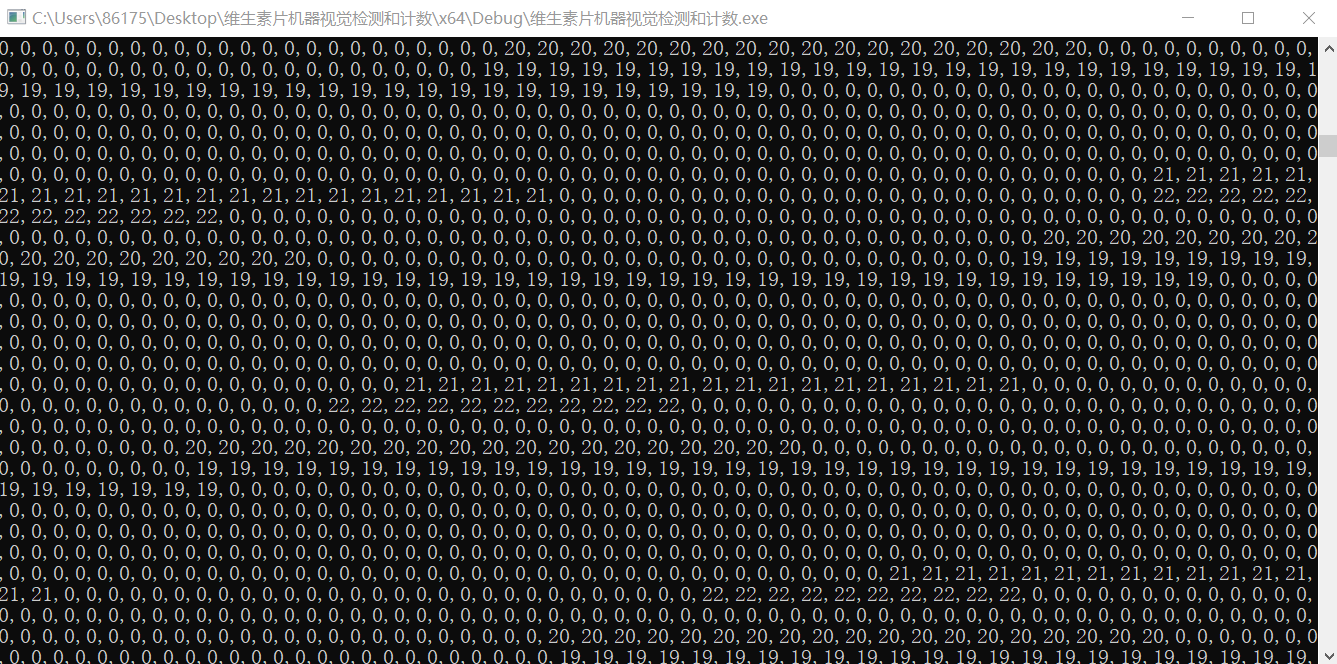（四）进行分水岭操作，并给分水岭后的区域随机上色，并打印出检测的药片个数。

 // 生成随机颜色
vector<Vec3b> colors;
for (size_t i = 0; i < contours.size(); i++) {
int r = theRNG().uniform(0, 255);
int g = theRNG().uniform(0, 255);
int b = theRNG().uniform(0, 255);
colors.push_back(Vec3b((uchar)b, (uchar)g, (uchar)r));
}

// 颜色填充与最终显示
Mat dst1 = Mat::zeros(markers.size(), CV_8UC3);
int index = 0;
for (int row = 0; row < markers.rows; row++) {
for (int col = 0; col < markers.cols; col++) {
index = markers.at<int>(row, col);

if (index > 0 && index <= contours.size()) {
dst1.at<Vec3b>(row, col) = colors[index - 1];

}
else {
dst1.at<Vec3b>(row, col) = Vec3b(0, 0, 0);
}

}
}
imshow("结果显示", dst1);
printf("药片检测个数: %d\n", contours.size());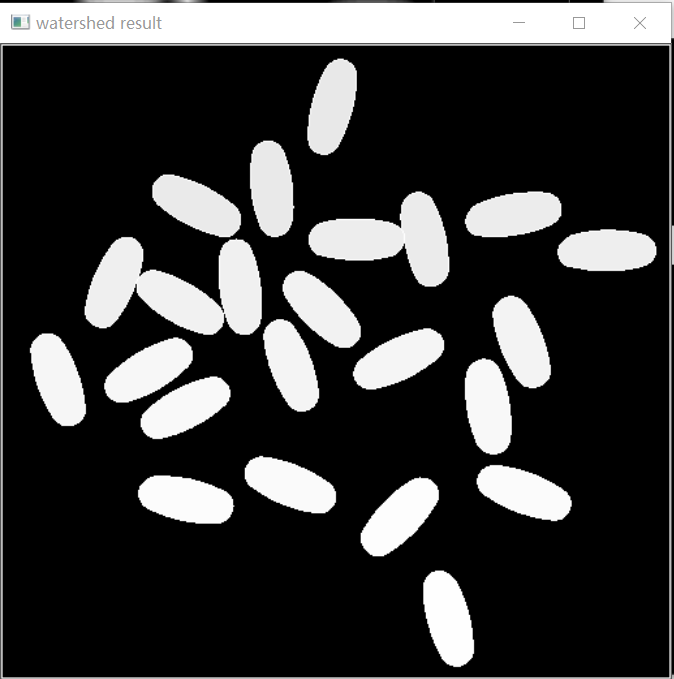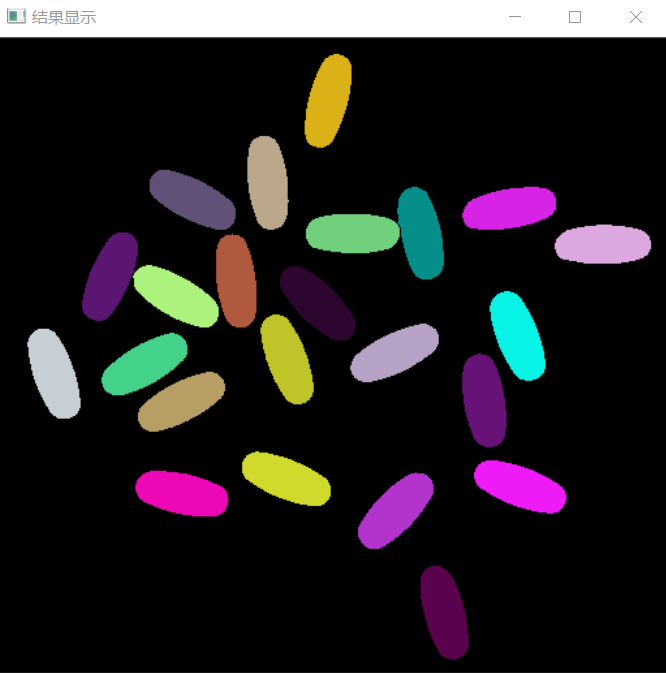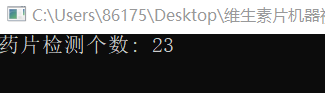🧡💛💚💙💜🤎🖤

（一）读入图像，形态学，二值化（消除噪声）

 Mat foreground, background, unkonwn;//创建前景，背景,未知区域
Mat src, src_binary, dst, src_distance;
imshow("原图片", src);
Mat kernel = getStructuringElement(MORPH_RECT, Size(3, 3), Point(-1, -1));
morphologyEx(src, dst, MORPH_OPEN, kernel);
imshow("形态学", dst);
cvtColor(dst, dst, COLOR_RGB2GRAY);
threshold(dst, src_binary, 100, 255, THRESH_OTSU);
imshow("二值化", src_binary);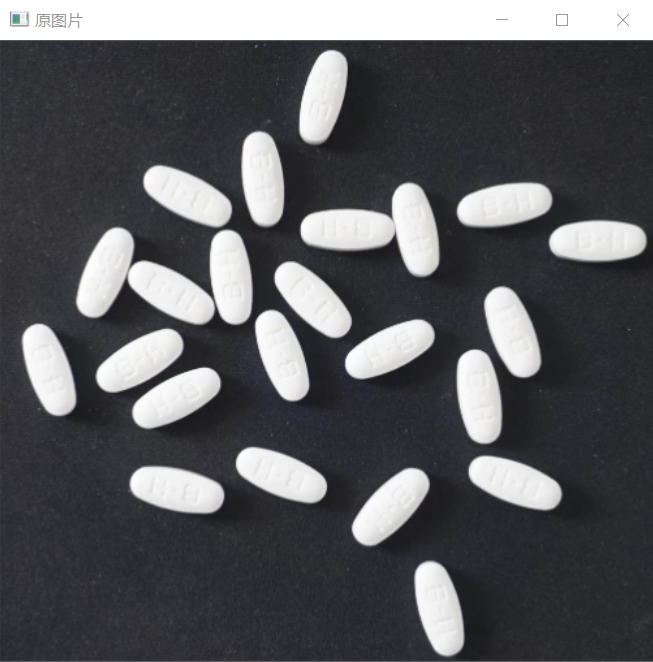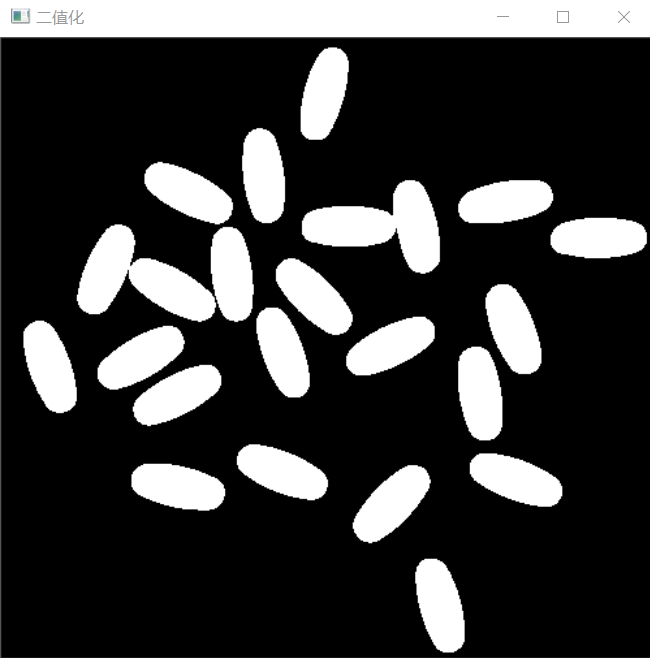（二）对二值化图像进行膨胀操作，得到大部分是背景的图片

//得到背景图片
dilate(src_binary, background, kernel, Point(-1, -1), 3);
imshow("背景图片", background);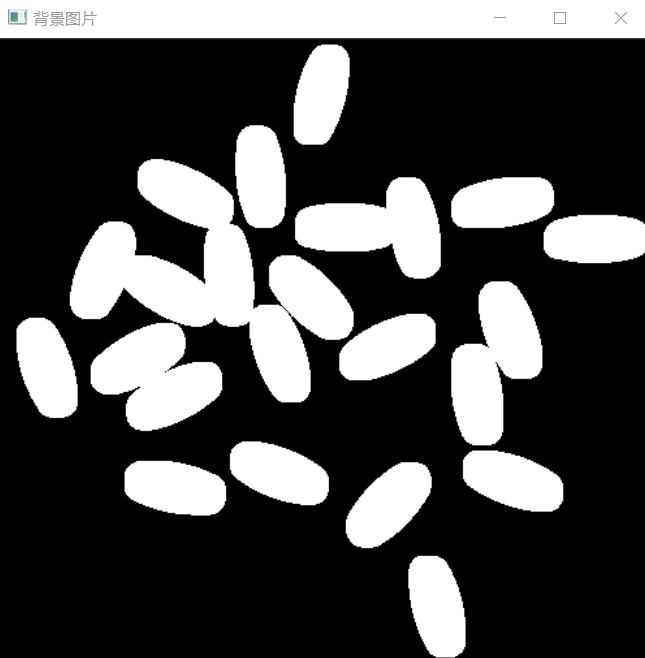（三）通过对二值图像距离变换得到前景图片（即注水点）

//距离变换
distanceTransform(src_binary, src_distance, DIST_L2, 3, 5);
imshow("距离变换", src_distance);
normalize(src_distance, src_distance, 0, 255, NORM_MINMAX);
double my_minv = 0.0, my_maxv = 0.0;
minMaxIdx(src_binary, &my_minv, &my_maxv);
threshold(src_distance, foreground, 0.4 * my_maxv, 255, THRESH_BINARY);
foreground.convertTo(foreground, CV_8U);
imshow("前景图片", foreground);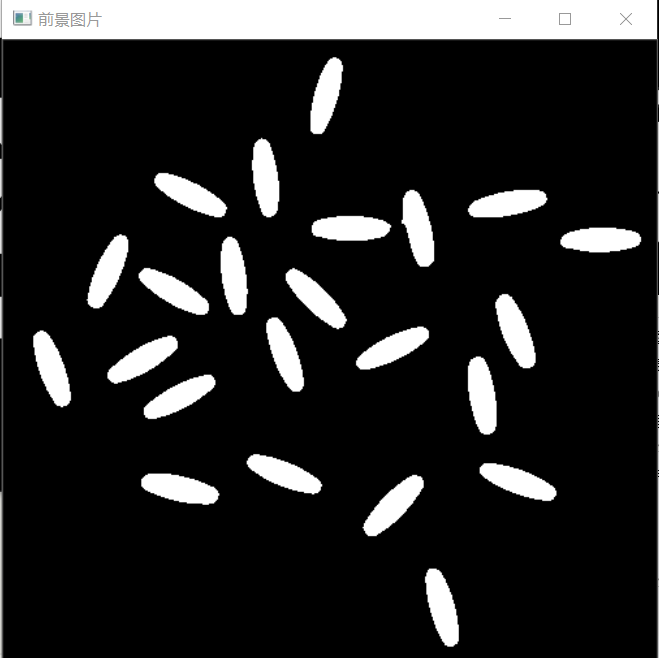（四）通过背景与前景的差值，得到未知区域（即边缘所在区域）

//得到未知区域
unkonwn = background - foreground;
imshow("未知区域", unkonwn);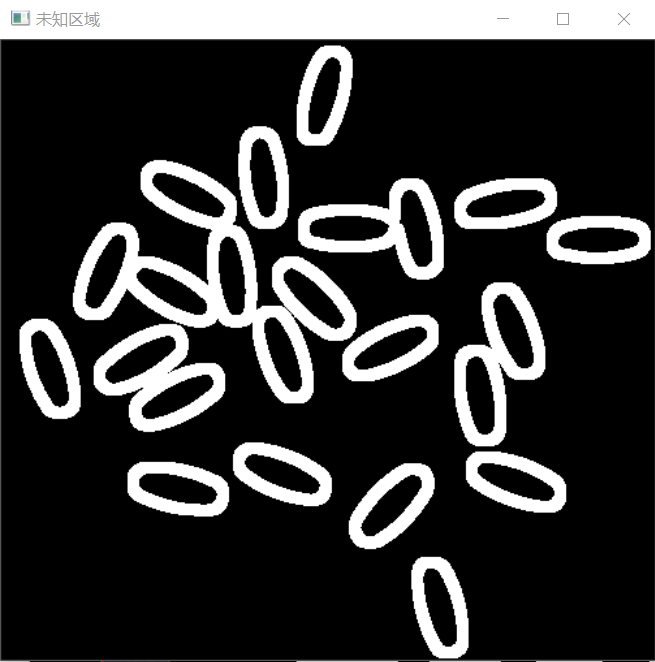（五）得到这些区域以后，我们可以获取注水点的标签，通过connectedComponents实现（即获取markers标签）

//创建标记点markers
Mat markers = Mat(src.size(), CV_32S);
int num = connectedComponents(foreground, markers, 8);
cout << num << endl;
markers = markers + 1;
for (int i = 0; i < unkonwn.rows; i++)
{
for (int j = 0; j < unkonwn.cols; j++)
{
if (((int)unkonwn.at<uchar>(i, j)) == 255)
{
markers.at<signed int>(i, j) = 0;
}
}
}

（六）进行分水岭操作，并显示边缘

watershed(src, markers);
for (int row = 0; row < markers.rows; row++)
{
for (int col = 0; col < markers.cols; col++)
{

if (markers.at< int>(row, col) == -1)
{
src.at<Vec3b>(row, col) = Vec3b(0, 0, 255);
}
}
}

imshow("结果", src);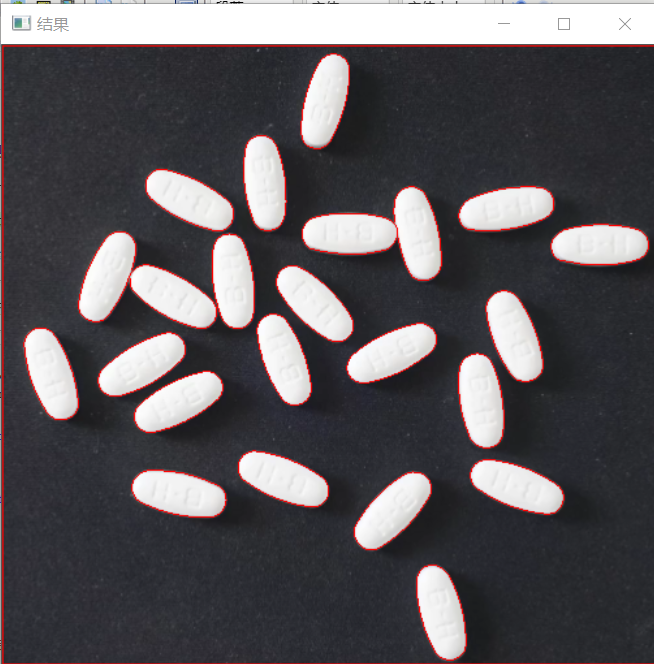posted @ 2021-05-17 16:12  唯有自己强大  阅读(1292)  评论(2编辑  收藏  举报